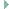board.h File Reference

Board specific definitions for the ST B-L072Z-LRWAN1 board. More...

## Detailed Description

Board specific definitions for the ST B-L072Z-LRWAN1 board.

Definition in file board.h.

`#include <stdint.h>`
`#include "cpu.h"`Include dependency graph for board.h:This graph shows which files directly or indirectly include this file:

Go to the source code of this file.

## Macros

#define BTN_B1_PIN   GPIO_PIN(PORT_B, 2)
User button.

## Functions

void board_init (void)
Initialize board specific hardware, including clock, LEDs and std-IO.

## xtimer configuration

#define XTIMER_WIDTH   (16)

## sx1276 configuration

#define SX127X_PARAM_SPI   (SPI_DEV(1))

#define SX127X_PARAM_SPI_NSS   GPIO_PIN(PORT_A, 15)

#define SX127X_PARAM_RESET   GPIO_PIN(PORT_C, 0)

#define SX127X_PARAM_DIO0   GPIO_PIN(PORT_B, 4)

#define SX127X_PARAM_DIO1   GPIO_PIN(PORT_B, 1)

#define SX127X_PARAM_DIO2   GPIO_PIN(PORT_B, 0)

#define SX127X_PARAM_DIO3   GPIO_PIN(PORT_C, 13)

#define RADIO_TCXO_VCC_PIN   GPIO_PIN(PORT_A, 12)

## LED pin definitions and handlers

#define LED0_PIN   GPIO_PIN(PORT_A, 5)

#define LED0_MASK   (1 << 5)

#define LED0_ON   (GPIOA->BSRR = LED0_MASK)

#define LED0_OFF   (GPIOA->BSRR = (LED0_MASK << 16))

#define LED0_TOGGLE   (GPIOA->ODR ^= LED0_MASK)

#define LED1_PIN   GPIO_PIN(PORT_B, 5)

#define LED1_MASK   (1 << 5)

#define LED1_ON   (GPIOB->BSRR = LED1_MASK)

#define LED1_OFF   (GPIOB->BSRR = (LED1_MASK << 16))

#define LED1_TOGGLE   (GPIOB->ODR ^= LED1_MASK)

#define LED2_PIN   GPIO_PIN(PORT_B, 6)

#define LED2_MASK   (1 << 6)

#define LED2_ON   (GPIOB->BSRR = LED2_MASK)

#define LED2_OFF   (GPIOB->BSRR = (LED2_MASK << 16))

#define LED2_TOGGLE   (GPIOB->ODR ^= LED2_MASK)

#define LED3_PIN   GPIO_PIN(PORT_B, 7)

#define LED3_MASK   (1 << 7)

#define LED3_ON   (GPIOB->BSRR = LED3_MASK)

#define LED3_OFF   (GPIOB->BSRR = (LED3_MASK << 16))

#define LED3_TOGGLE   (GPIOB->ODR ^= LED3_MASK)